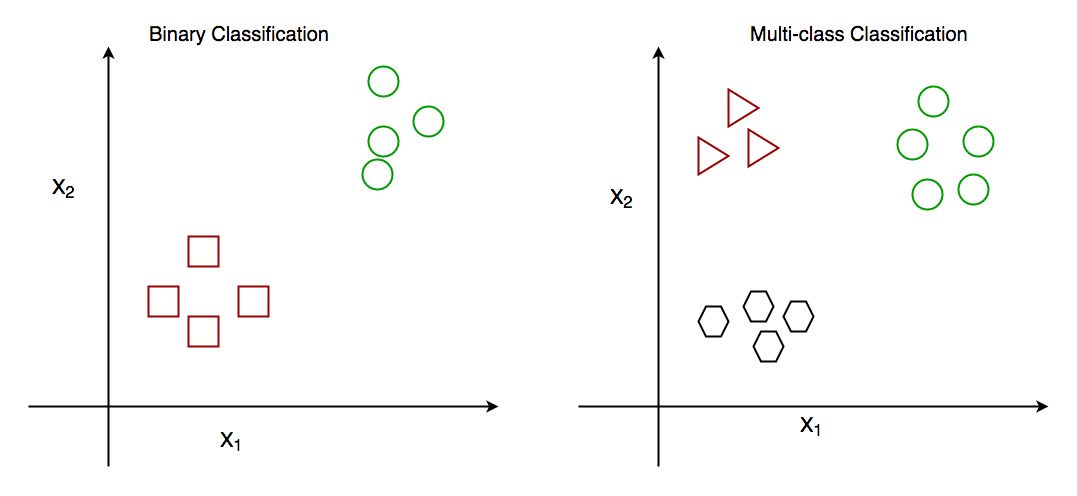# One-vs-Rest strategy for Multi-Class Classification

Prerequisite: Getting Started with Classification/

Classification is perhaps the most common Machine Learning task. Before we jump into what One-vs-Rest (OVR) classifiers are and how they work, you may follow the link below and get a brief overview of what classification is and how it is useful.

In general, there are two types of classification algorithms:

1. Binary classification algorithms.
2. Multi-class classification algorithms.

Binary classification is when we have to classify objects into two groups. Generally, these two groups consist of ‘True’ and ‘False’. For example, given a certain set of health attributes, a binary classification task may be to determine whether a person has diabetes or not.

On the other hand, in multi-class classification, there are more than two classes. For example, given a set of attributes of fruit, like it’s shape and colour, a multi-class classification task would be to determine the type of fruit.So, now that you have an idea of how binary and multi-class classification work, let us get on to how the one-vs-rest heuristic method is used.

One-vs-Rest (OVR) Method:
Many popular classification algorithms were designed natively for binary classification problems. These algorithms include :

• Logistic Regression
• Support Vector Machines (SVM)
• Perceptron Models

and many more.

So, these popular classification algorithms cannot directly be used for multi-class classification problems. Some heuristic methods are available that can split up multi-class classification problems into many different binary classification problems. To understand how this works, let us consider an example : Say, a classification problem is to classify various fruits into three types of fruits: banana, orange or apple. Now, this is clearly a multi-class classification problem. If you want to use a binary classification algorithm like, say SVM. The way One-vs-Rest method will deal with this is illustrated below :

Since there are three classes in the classification problem, the One-vs-Rest method will break down this problem into three binary classification problems:

• Problem 1 : Banana vs [Orange, Apple]
• Problem 2 : Orange vs [Banana, Apple]
• Problem 3 : Apple vs [Banana, Orange]

So instead of solving it as (Banana vs Orange vs Apple), it is solved using three binary classification problems as shown above.

A major downside or disadvantage of this method is that many models have to be created. For a multi-class problem with ‘n’ number of classes, ‘n’ number of models have to be created, which may slow down the entire process. However, it is very useful with datasets having a small number of classes, where we want to use a model like SVM or Logistic Regression.

Implementation of One-vs-Rest method using Python3
Python’s scikit-learn library offers a method OneVsRestClassifier(estimator, *, n_jobs=None) to implement this method. For this implementation, we will be using the popular ‘Wine dataset’, to determine the origin of wines using chemical attributes. We can direct this dataset using scikit-learn. To know more about this dataset, you can use the link below : Wine Dataset

We will use a Support Vector Machine, which is a binary classification algorithm and use it with the One-vs-Rest heuristic to perform multi-class classification.

To evaluate our model, we will see the accuracy score of the test set and the classification report of the model.

 `from` `sklearn.datasets ``import` `load_wine ` `from` `sklearn.multiclass ``import` `OneVsRestClassifier ` `from` `sklearn.svm ``import` `SVC ` `from` `sklearn.metrics ``import` `accuracy_score, classification_report ` `from` `sklearn.model_selection ``import` `train_test_split ` `import` `warnings ` `  `  `'''  ` `We are ignoring warnings because of a peculiar fact about this ` `dataset. The 3rd label, 'Label2' is never predicted and so the python  ` `interpreter throws a warning. However, this can safely be ignored because  ` `we are not concerned if a certain label is predicted or not  ` `'''` `warnings.filterwarnings(``'ignore'``) ` `  `  `# Loading the dataset ` `dataset ``=` `load_wine() ` `X ``=` `dataset.data ` `y ``=` `dataset.target ` `  `  `# Splitting the dataset into training and testing sets ` `X_train, X_test, y_train, y_test ``=` `train_test_split( ` `    ``X, y, test_size ``=` `0.1``, random_state ``=` `13``) ` `  `  `# Creating the SVM model ` `model ``=` `OneVsRestClassifier(SVC()) ` `  `  `# Fitting the model with training data ` `model.fit(X_train, y_train) ` `  `  `# Making a prediction on the test set ` `prediction ``=` `model.predict(X_test) ` `  `  `# Evaluating the model ` `print``(f"Test ``Set` `Accuracy : {accuracy_score( ` `    ``y_test, prediction) ``*` `100``} ``%``\n\n") ` `print``(f"Classification Report : \n\n{classification_report( ` `    ``y_test, prediction)}") `

Output:

```Test Set Accuracy : 66.66666666666666 %

Classification Report :

precision    recall  f1-score   support

0       0.62      1.00      0.77         5
1       0.70      0.88      0.78         8

micro avg       0.67      0.92      0.77        13
macro avg       0.66      0.94      0.77        13
weighted avg       0.67      0.92      0.77        13
```

We get a test set accuracy of approximately 66.667%. This is not bad for this dataset. This dataset is notorious for being difficult to classify and the benchmark accuracy is 62.4 +- 0.4 %. So, our result is actually quite good.

Conclusion:
Now that you know how to use the One-vs-Rest heuristic method for performing multi-class classification with binary classifiers, you can try using it next time you have to perform some multi-class classification task.

Attention geek! Strengthen your foundations with the Python Programming Foundation Course and learn the basics.

To begin with, your interview preparations Enhance your Data Structures concepts with the Python DS Course.

My Personal Notes arrow_drop_upLooking for a job as a software developer Passing out from college in August, 2021

If you like GeeksforGeeks and would like to contribute, you can also write an article using contribute.geeksforgeeks.org or mail your article to contribute@geeksforgeeks.org. See your article appearing on the GeeksforGeeks main page and help other Geeks.

Please Improve this article if you find anything incorrect by clicking on the "Improve Article" button below.

Article Tags :
Practice Tags :

Be the First to upvote.

Please write to us at contribute@geeksforgeeks.org to report any issue with the above content.